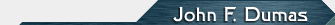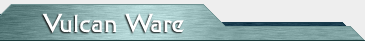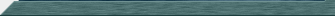Permute A template c++ class for generating all permutations of a set of 'N' items. Here's an example showing how the class is typically used: ``` template void show(Permute &p) { p.reset(); while(true) { const vector *current = p.getNext(); if(!current) break; cout << Permute::toString(current) << endl; } } static int main() { Permute pLetters("abcd"); show(pLetters); int numbers[] = { 1, 2, 3, 4 }; Permute pNumbers(numbers, 4); show(pNumbers); } ``` The output from the above would look like ... ``` a b c d b a c d c a b d a c b d b c a d c b a d d a b c a d b c b d a c ... 1 2 3 4 2 1 3 4 3 1 2 4 1 3 2 4 2 3 1 4 ... ``` The interesting thing about this class is that way the permutations are generated. The algorithm used is one I created myself and it works as follows: ``` Suppose we have a list containing the letters a, b, c, d Now, here are the first 10 permutations ... a b c d 0 a b d c 1 a c d b 2 a c b d 3 a d b c 4 a d c b 5 b c d a 6 b c a d 7 b d a c 8 b d c a 9 ... Notice that each permutation is produced by reversing a part of the previous permutation, for example: a b c d 0 a b d c 1 Here we're reversing the sub string "c d" to get from permutation 0 to permutation 1. Similarly ... a c b d 3 a d b c 4 a d c b 5 b c d a 6 Here, to get from 3 to 4 we're reversing the string "c b d", to get from 5 to 6 we're reversing "a d c b". Each time we're reversing the last n characters of the string, the pattern looks like this ... Last 'n' characters Permutation ------------------- ----------- N/A 0 2 1 3 2 2 3 3 4 2 5 4 6 2 7 3 8 2 9 ... Now, for each permutation number { 1, ..., 23 } we find the largest factorial value that divides evenly into the permutation number. The factorial values less than 23 are: 1! -> 1 2! -> 2 3! -> 6 permutation number biggest divisor reverse last n chars ------------------ --------------- -------------------- 4 2! 3 5 1! 2 6 3! 4 ```Source CodeReturn to Main